# DPL 9 Help

Uniform Distribution

#Uniform Distribution

The Uniform Distribution is useful for an uncertainty whose range can be identified but for which there is no basis for concluding that any values within the range are more likely than any others. In general terms, it is the basis for defining a range from which a random number will be generated. It may be useful when describing flow, such as the distribution of traffic along a straight road (in any given interval there is a probability p of a vehicle being there). In error analysis it is used to represent the distribution of rounding-off errors or corrections for grouping.

The Uniform Distribution is also used in failure analysis when dealing with life-testing. Some examples of this would be the sum of the earliest failure times, the total observed life up to the nth failure, or the sum of all n failure times.

The Uniform Distribution represents an equal chance of any value between min and max occurring. The parameters of this distribution are min and max, which can have any values as long as max is larger than min. The distribution is: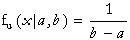where min<x<max.

The equations for the mean and variance are: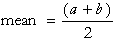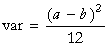The equations for the parameters are: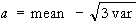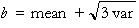Versions: DPL Professional, DPL Enterprise, DPL Portfolio